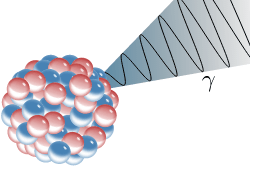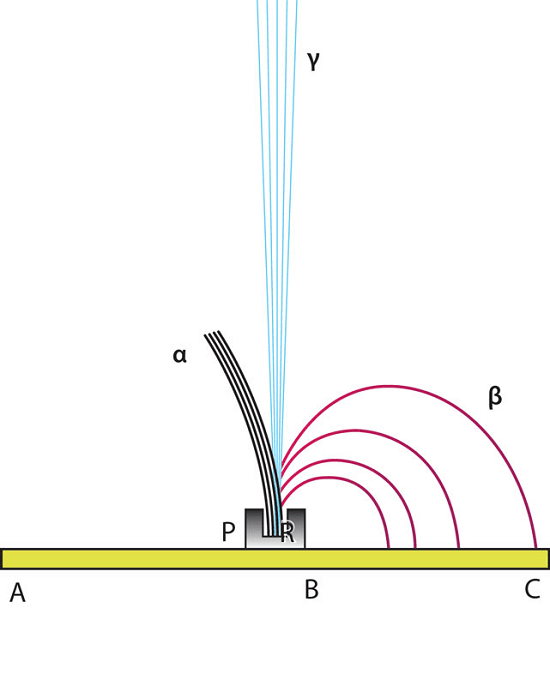# Difference Between Alpha Beta and Gamma Radiation

Alpha, beta and gamma radiation are three different types of nuclear radiation. These three different types of radiation have different properties. Here, we discuss the difference between alpha, beta and gamma radiation. Their basic properties and differences were discussed in the article “What are the Three Types of Nuclear Radiation“.

## Alpha Beta and Gamma Particles

• Alpha radiation consists of alpha particles or helium nuclei ($_{2}^{4}\mathrm{He}$), that is, a particle of alpha radiation consists of two protons and two neutrons bound together.• Beta radiation could refer to either beta minus radiation, where electrons ($e^-$) are emitted or beta plus radiation, where positrons ($e^+$) are emitted.• Gamma radiation refers to the radiation of an electromagnetic wave in the gamma range. It can also be considered to be a photon ($\gamma$).## Charge of Alpha Beta and Gamma Radiation

• Alpha particles have a charge of ($\mathbf{+2e}$) from its two protons, where$e=\mathrm{1.6\times 10^{-19}\:C}$.
• For beta radiation, an electron has a charge of$\mathbf{-e}$. A positron, being the antiparticle of the electron, has a charge of$\mathbf{+e}$.
• Photons which carry gamma radiation are not charged.

## Mass of Alpha Beta and Gamma Radiation

• Alpha particles are made of four nucleons. Therefore, they have a mass of approximately$4\mathrm{\:u}$, where$\mathrm{u=1.66\times 10^{-27}\:kg}$. So, the mass of an alpha particle is 6.64×10-27 kg = 3.73 GeV/c2.
• Electrons and Positrons, which make up beta particles, are antiparticles of each other. This means that they both have the same mass. The mass of an electron/positron is 5.49×10-4 u  = 6.64×10-27 kg = 511 keV/c2.
• Photons are massless, so gamma radiation carry no mass.

## Speed of Alpha Beta and Gamma Radiation

• Alpha decay only takes place when masses of the daughter nucleus ($M_D$) and the alpha particle together ($M_{\alpha}$) is less than the mass of the parent nucleus ($M_P$). The difference in masses ($M_P-M_D-M_{\alpha}$) is given as kinetic energy to both the alpha particle and the daughter nucleus.To conserve momentum, the daughter nucleus and the alpha particle must travel in opposite directions. In addition, the alpha particle, being much lighter than the typical daughter nucleus, carries off most kinetic energy (again, to conserve momentum). Typically, the alpha particles have speeds of about 5% the speed of light ($\mathbf{0.05c}$, where$c=$ speed of light = 3×108 m s-1. For a given alpha decay the kinetic energy, and therefore the speed of the alpha particle, takes a specific value which can be calculated from the mass differences in nuclei and from the law of conservation of momentum.
• For beta decay, there are three products that share the kinetic energy available. In this case, the kinetic energy may be shared between particles in any way. As a result, the beta particles can take a range of values. Typically, they take on values of up to$\mathbf{0.99c}$.
• Gamma radiation consists of photons. They travel at the speed of light,$\mathbf{c}$. They do carry specific energies, however, corresponding to the specific transition of nuclear energy levels that caused them to be emitted.

## Ionising Power of Alpha Beta and Gamma Radiation

• Alpha particles can produce about 1 000 000 pairs of ions per centimetre as they travel through the air. This is relatively high. This is because they have a relatively large mass and they move slowly, allowing them to interact more with air molecules.
• Beta particles produce about 10 000 pairs of ions per centimetre in air.
• Gamma rays (photons) can produce about 10 pairs of ions per centimetre in air.

## Effect of Magnetic Field on Alpha Beta and Gamma Radiation

• Alpha particles have a charge, so if a magnetic field is applied perpendicular to its path, the alpha particle shows some deflection.
• Beta particles also have a charge. Compared to alpha particles, beta particles’ charge is half that of an alpha particles’ charge. On the other hand, speeds of beta particles are much larger than those of alpha. As a result, beta particles are deflected more strongly by magnetic fields applied perpendicular to their paths. When placed under the same magnetic field, a beta minus particle bends in the opposite direction to that of an alpha particle, while a beta plus particle would bend in the same direction as does the alpha particle.
• Photons are not charged and so they do not get deflected by magnetic fields.From Marie Curie’s Demonstration of the Behavior of 3 Types of Radiation

## Ability to Stop Alpha Beta and Gamma Radiation

• Alpha particles are strongly ionising. So as they travel through matter, they lose their energy much more quickly. Hence, they can be stopped easily. Alpha particles can travel a few centimetres in the air before they are stopped. They can also be stopped by a thick piece of paper. They cannot penetrate through human skin, either, so they are not as dangerous, so long as they remain outside our bodies. Once they are inside the body, they can cause much more damage than beta and gamma since they have a much stronger ability to ionise. (In a famous case, Alexander Litvinenko, a former Russian secret agent, is believed to have been deliberately poisoned with polonium-210, an alpha emitter. It is also difficult to detect alpha particles since they cannot leave the body once they are inside. However, traces of alpha radiation had been found where he had used public restrooms).
• Beta particles can travel several metres in the air, but they can be stopped by a sheet of aluminium several millimetres thick.
• Gamma photons interact the least with matter, and consequently they are much harder to stop. Several centimetres of lead or a few metres of concrete is needed to appreciably reduce the intensity of gamma radiation.## Difference Between Alpha Beta and Gamma Radiation – Summary

 Property Alpha radiation Beta radiation Gamma radiation Nature of particle A helium nucleus An electron/positron A photon Charge$+2e$$\pm e$ 0 Mass$4\mathrm{u}$$\sim\frac{\mathrm{u}}{2000}$ 0 Speed ~0.05c up to 0.99c c Ion pairs per cm of air ~1 000 000 ~10 000 ~10 Interaction with perpendicular magnetic fields Some deflection Large defleciton No deflection Stopped by Thick sheet of paper Few mm of aluminium sheet (to some extent) A couple of cm of a block of lead or few metres thick concrete

###### “Penetration of Alpha Beta Gamma Radiation” By Alfa_beta_gamma_radiation.svg: User:Stannered derivative work: Ehamberg (Alfa_beta_gamma_radiation.svg) [CC BY 2.5 , CC-BY-SA-3.0 or GFDL], via Wikimedia Commons